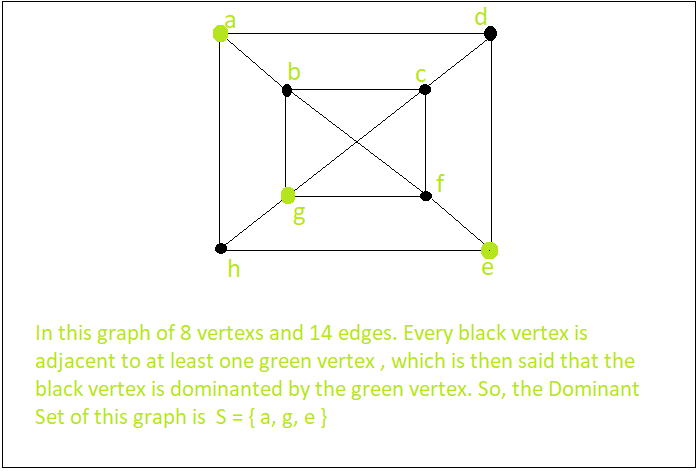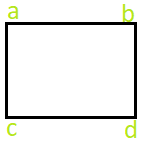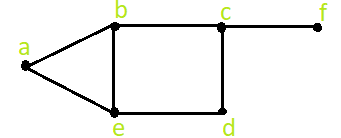# Dominant Set of a Graph

In graph theory, a dominating set for a graph G = (V, E) is a subset D of V such that every vertex not in D is adjacent to at least one member of D. The domination number is the number of vertices in a smallest dominating set for G.Examples:

```Input :   A graph with 4 vertex and 4 edgesOutput :  The Dominant Set S= { a, b } or { a, d } or { a, c } and more.

Input : A graph with 6 vertex and 7 edgesOutput :  The Dominant Set S= { a, d, f } or { e, c } and more.
```

## Recommended: Please try your approach on {IDE} first, before moving on to the solution.

It is believed that there may be no efficient algorithm that finds a smallest dominating set for all graphs, but there are efficient approximation algorithms.
Algorithm :

• First we have to initialize a set ‘S’ as empty
• Take any edge ‘e’ of the graph connecting the vertices ( say A and B )
• Add one vertex between A and B ( let say A ) to our set S
• Delete all the edges in the graph connected to A
• Go back to step 2 and repeat, if some edge is still left in the graph
• The final set S is a Dominant Set of the graph

## C++

 `// C++ program to find the Dominant Set of a graph ` `#include ` `using` `namespace` `std; ` ` `  `vector > g; ` `bool` `box; ` ` `  `vector<``int``> Dominant(``int` `ver, ``int` `edge) ` `{ ` `    ``vector<``int``> S; ``// set S ` `    ``for` `(``int` `i = 0; i < ver; i++) { ` `        ``if` `(!box[i]) { ` `            ``S.push_back(i); ` `            ``box[i] = ``true``; ` `            ``for` `(``int` `j = 0; j < (``int``)g[i].size(); j++) { ` `                ``if` `(!box[g[i][j]]) { ` `                    ``box[g[i][j]] = ``true``; ` `                    ``break``; ` `                ``} ` `            ``} ` `        ``} ` `    ``} ` `    ``return` `S; ` `} ` ` `  `// Driver function ` `int` `main() ` `{ ` `    ``int` `ver, edge, x, y; ` ` `  `    ``ver = 5; ``// Enter number of vertices ` `    ``edge = 6; ``// Enter number of Edges ` `    ``g.resize(ver); ` ` `  `    ``// Setting all index value of an array as 0 ` `    ``memset``(box, 0, ``sizeof``(box));  ` ` `  `    ``// Enter all the end-points of all the Edges ` `    ``// g[x--].push_back[y--]      g[y--].push_back[x--] ` `    ``g.push_back(1); ` `    ``g.push_back(0); ``// x = 1, y = 2 ; ` `    ``g.push_back(2); ` `    ``g.push_back(1); ``// x = 2, y = 3 ; ` `    ``g.push_back(3); ` `    ``g.push_back(2); ``// x = 3, y = 4 ; ` `    ``g.push_back(3); ` `    ``g.push_back(0); ``// x = 1, y = 4 ; ` `    ``g.push_back(4); ` `    ``g.push_back(3); ``// x = 4, y = 5 ; ` `    ``g.push_back(4); ` `    ``g.push_back(2); ``// x = 3, y = 5 ; ` ` `  `    ``vector<``int``> S = Dominant(ver, edge); ` `    ``cout << ``"The Dominant Set is : { "``; ` `    ``for` `(``int` `i = 0; i < (``int``)S.size(); i++) ` `        ``cout << S[i] + 1 << ``" "``; ` `    ``cout << ``"}"``; ` `    ``return` `0; ` `} `

## Java

 `// Java program to find the Dominant Set of a graph ` `import` `java.util.*; ` ` `  `class` `GFG ` `{ ` ` `  `static` `Vector []g; ` `static` `boolean` `[]box = ``new` `boolean``[``100000``]; ` ` `  `static` `Vector Dominant(``int` `ver, ``int` `edge) ` `{ ` `    ``Vector S = ``new` `Vector(); ``// set S ` `    ``for` `(``int` `i = ``0``; i < ver; i++)  ` `    ``{ ` `        ``if` `(!box[i])  ` `        ``{ ` `            ``S.add(i); ` `            ``box[i] = ``true``; ` `            ``for` `(``int` `j = ``0``; j < (``int``)g[i].size(); j++)  ` `            ``{ ` `                ``if` `(!box[g[i].get(j)]) ` `                ``{ ` `                    ``box[g[i].get(j)] = ``true``; ` `                    ``break``; ` `                ``} ` `            ``} ` `        ``} ` `    ``} ` `    ``return` `S; ` `} ` ` `  `// Driver code ` `public` `static` `void` `main(String[] args) ` `{ ` `    ``int` `ver, edge, x, y; ` ` `  `    ``ver = ``5``; ``// Enter number of vertices ` `    ``edge = ``6``; ``// Enter number of Edges ` `    ``g = ``new` `Vector[ver]; ` `    ``for` `(``int` `i = ``0``; i < ver; i++) ` `        ``g[i] = ``new` `Vector(); ` ` `  ` `  `    ``// Enter all the end-points of all the Edges ` `    ``// g[x--].push_back[y--]     g[y--].push_back[x--] ` `    ``g[``0``].add(``1``); ` `    ``g[``1``].add(``0``); ``// x = 1, y = 2 ; ` `    ``g[``1``].add(``2``); ` `    ``g[``2``].add(``1``); ``// x = 2, y = 3 ; ` `    ``g[``2``].add(``3``); ` `    ``g[``3``].add(``2``); ``// x = 3, y = 4 ; ` `    ``g[``0``].add(``3``); ` `    ``g[``3``].add(``0``); ``// x = 1, y = 4 ; ` `    ``g[``3``].add(``4``); ` `    ``g[``4``].add(``3``); ``// x = 4, y = 5 ; ` `    ``g[``2``].add(``4``); ` `    ``g[``4``].add(``2``); ``// x = 3, y = 5 ; ` ` `  `    ``Vector S = Dominant(ver, edge); ` `    ``System.out.print(``"The Dominant Set is : { "``); ` `    ``for` `(``int` `i = ``0``; i < (``int``)S.size(); i++) ` `        ``System.out.print(S.get(i) + ``1` `+ ``" "``); ` `    ``System.out.print(``"}"``); ` `} ` `} ` ` `  `// This code is contributed by Rajput-Ji `

## C#

 `// C# program to find the Dominant Set of a graph ` `using` `System; ` `using` `System.Collections.Generic; ` ` `  `class` `GFG ` `{ ` ` `  `static` `List<``int``> []g; ` `static` `bool` `[]box = ``new` `bool``; ` ` `  `static` `List<``int``> Dominant(``int` `ver, ``int` `edge) ` `{ ` `    ``List<``int``> S = ``new` `List<``int``>(); ``// set S ` `    ``for` `(``int` `i = 0; i < ver; i++)  ` `    ``{ ` `        ``if` `(!box[i])  ` `        ``{ ` `            ``S.Add(i); ` `            ``box[i] = ``true``; ` `            ``for` `(``int` `j = 0; j < (``int``)g[i].Count; j++)  ` `            ``{ ` `                ``if` `(!box[g[i][j]]) ` `                ``{ ` `                    ``box[g[i][j]] = ``true``; ` `                    ``break``; ` `                ``} ` `            ``} ` `        ``} ` `    ``} ` `    ``return` `S; ` `} ` ` `  `// Driver code ` `public` `static` `void` `Main(String[] args) ` `{ ` `    ``int` `ver, edge; ` ` `  `    ``ver = 5; ``// Enter number of vertices ` `    ``edge = 6; ``// Enter number of Edges ` `    ``g = ``new` `List<``int``>[ver]; ` `    ``for` `(``int` `i = 0; i < ver; i++) ` `        ``g[i] = ``new` `List<``int``>(); ` ` `  `    ``// Enter all the end-points of all the Edges ` `    ``// g[x--].push_back[y--]     g[y--].push_back[x--] ` `    ``g.Add(1); ` `    ``g.Add(0); ``// x = 1, y = 2 ; ` `    ``g.Add(2); ` `    ``g.Add(1); ``// x = 2, y = 3 ; ` `    ``g.Add(3); ` `    ``g.Add(2); ``// x = 3, y = 4 ; ` `    ``g.Add(3); ` `    ``g.Add(0); ``// x = 1, y = 4 ; ` `    ``g.Add(4); ` `    ``g.Add(3); ``// x = 4, y = 5 ; ` `    ``g.Add(4); ` `    ``g.Add(2); ``// x = 3, y = 5 ; ` ` `  `    ``List<``int``> S = Dominant(ver, edge); ` `    ``Console.Write(``"The Dominant Set is : { "``); ` `    ``for` `(``int` `i = 0; i < (``int``)S.Count; i++) ` `        ``Console.Write(S[i] + 1 + ``" "``); ` `    ``Console.Write(``"}"``); ` `} ` `} ` ` `  `// This code is contributed by PrinciRaj1992 `

Output:

```The Dominant Set is : { 1 3 5 }
```

Reference : wiki

Don’t stop now and take your learning to the next level. Learn all the important concepts of Data Structures and Algorithms with the help of the most trusted course: DSA Self Paced. Become industry ready at a student-friendly price.

My Personal Notes arrow_drop_upCheck out this Author's contributed articles.

If you like GeeksforGeeks and would like to contribute, you can also write an article using contribute.geeksforgeeks.org or mail your article to contribute@geeksforgeeks.org. See your article appearing on the GeeksforGeeks main page and help other Geeks.

Please Improve this article if you find anything incorrect by clicking on the "Improve Article" button below.

Improved By : Rajput-Ji, princiraj1992

Article Tags :
Practice Tags :

1

Please write to us at contribute@geeksforgeeks.org to report any issue with the above content.